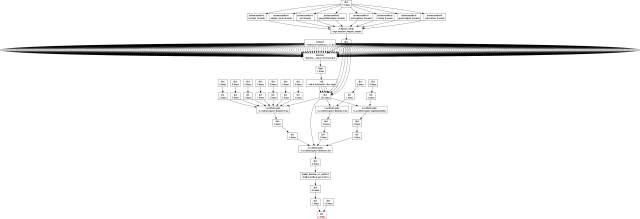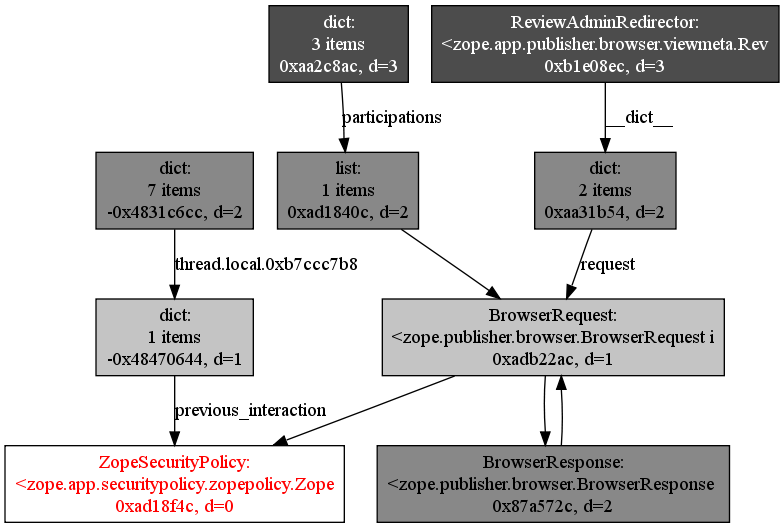This is a continuation of Python object graphs.

```import gc
import inspect
import types
import weakref
import os
```

I've already mentioned gc and inspect. types and weakref help me distinguish certain kinds of objects. os is needed to spawn dot to generate an image from the textual graph description.

```def show_backrefs(objs, max_depth=3, extra_ignore=(), filter=None, too_many=10):
```

This function starts from a given object (or a list of objects) and follows the reference chains backwards, up to a given maximal depth.

The extra_ignore argument is the same as for find_backref_chain, which I explained earlier.

The filter argument serves the same function, but instead of explicitly listing all the id of the objects I want to ignore here I can pass a function that takes an object and returns True if that object is interesting. This would be useful if I wanted to ignore all objects of a certain class, but it turned out I actually didn't need this.

Finally, too_many defines the branching factor after which new extra references to the same object would just clutter the graph too much to be useful.

```    if not isinstance(objs, (list, tuple)):
objs = [objs]
```

For convenience, I accept either a single object, or a list of objects.

```    f = file('objects.dot', 'w')
```

It's not nice to hardcode filenames, but this is debugging code. Different quality standards apply.

```    print >> f, 'digraph ObjectGraph {'
print >> f, '  node[shape=box, style=filled, fillcolor=white];'
```

This is what graphviz files look like. I'm setting up some attribute defaults.

```    queue = []
depth = {}
```

The standard BFS elements again.

```    ignore = set(extra_ignore)
```

Don't want the graphing code implementation to contaminate the graph itself.

```    for obj in objs:
print >> f, '  %s[fontcolor=red];' % (obj_node_id(obj))
depth[id(obj)] = 0
queue.append(obj)
```

Initialize the BFS data structures with our start points as well as making sure they are highlighted in red in the graph description file. I'll show the helper functions such as obj_node_id after the main function.

```    gc.collect()
```

Just to make sure we have no collectable garbage lying around.

```    nodes = 0
while queue:
nodes += 1
target = queue.pop(0)
```

The standard BFS loop

```        tdepth = depth[id(target)]
print >> f, '  %s[label="%s"];' % (obj_node_id(target), obj_label(target, tdepth))
```

Nodes have labels, obviously.

```        h, s, v = gradient((0, 0, 1), (0, 0, .3), tdepth, max_depth)
if inspect.ismodule(target):
h = .3
s = 1
print >> f, '  %s[fillcolor="%g,%g,%g"];' % (obj_node_id(target), h, s, v)
```

Make the background color depend on the depth of an object, in a gradient from white to dark gray. Except for modules, which we want to highlight in green (hue 30% or 108°, saturation 100%, value inherited from the gradient). Modules show the root source of a memory leak, in my case1, and I want them to be easily noticeable.

1 Other possibilities for leaks include having a reference to an object from a call-stack frame, or having a loop of objects with __del__ methods, or a bug in a C extension module.
```        if tdepth == max_depth:
print >> f, '  %s[fontcolor=white];' % (obj_node_id(target))
```

The objects farthest away have the darkest background. Switching to white font color improves contrast, lets me use a wider gradient, and also acts as a reminder that the graph is incomplete — truncated at these nodes.

```        if inspect.ismodule(target) or tdepth >= max_depth:
continue
```

Never look up references to modules -- we already found what we wanted, extra backreferences would just clutter up the graph. Also stop if we've reached the maximum desired depth.

```        referrers = gc.get_referrers(target)
```

We don't want new objects created during the search to become part of the graph.

```        n = 0
for source in referrers:
if inspect.isframe(source) or id(source) in ignore:
continue
if filter and not filter(source):
continue
```

Ignore objects we were told to ignore, as well as stack frames (for convenience).

```            elabel = edge_label(source, target)
print >> f, '  %s -> %s%s;' % (obj_node_id(source), obj_node_id(target), elabel)
```

Add the edge to the graph, with an optional label. By the way, I really like the graph description language.

```            if id(source) not in depth:
depth[id(source)] = tdepth + 1
queue.append(source)
```

Standard BFS steps.

```            n += 1
if n >= too_many:
print >> f, '  %s[color=red];' % obj_node_id(target)
break
```

If we have too many references to a single object, avoid the Starship Enterprise graph2, change the border color to red, and skip all further references to this particular object. This is lossy, but it's better than to get overwhelmed with too much information.

2 Here's what it looks like:It was produced by an older prototype that didn't have coloured backgrounds.
```    print >> f, "}"
f.close()
print "Graph written to objects.dot (%d nodes)" % nodes
```

Ta dah!

```    os.system("dot -Tpng objects.dot > objects.png")
print "Image generated as objects.png"
```

If you want to, you might start an image viewer too at this point.

Now the helper functions:

```def obj_node_id(obj):
if isinstance(obj, weakref.ref):
return 'all_weakrefs_are_one'
return ('o%d' % id(obj)).replace('-', '_')
```

Every graph node must have an alphanumeric ID in a graphviz dot file. The ID cannot start with a number, and cannot contain minus signs, so we massage ids a bit.

The special case for weakref.ref objects is here because I once had a graph with 300 of them, and they each had exactly one reference and one backreference. It was a lot of clutter for no value, so I thought I'd merge them to a single node. Later I saw this wasn't enough (see the Starship Enterprise above) and added the too_many limit.

```def obj_label(obj):
return quote(type(obj).__name__ + ':\n' +
safe_repr(obj) + '\n' +
hex(id(obj)) + ', d=%d' % depth)
```

I show four things in the nodes: the type of the object, a short representation of the object, its id and its depth. In retrospect, I think the last two don't add enough value to pay for the space they take up.

```def quote(s):
return s.replace("\\", "\\\\").replace("\"", "\\\"").replace("\n", "\\n")
```

Newlines, double quotes, and backslashes inside strings confuse graphviz and must be escaped.

```def safe_repr(obj):
try:
return short_repr(obj)
except:
return '(unrepresentable)'
```

Generating object graphs is time-consuming, and it's not fun when a bug in you custom repr() function causes it to fail.

```def short_repr(obj):
if isinstance(obj, (type, types.ModuleType, types.BuiltinMethodType,
types.BuiltinFunctionType)):
return obj.__name__
```

This is going to be the pattern of this function: a series of if statements. I use obj.__name__ instead of repr() or str() because the graph nodes already show the type of obj. Repeating the same information and showing "type: <type 'str'>" instead of "type: str" would be redundant and would make the graph nodes needlessly big.

```    if isinstance(obj, types.MethodType):
if obj.im_self is not None:
return obj.im_func.__name__ + ' (bound)'
else:
return obj.im_func.__name__
```

Distinguishing bound and unbound methods is a nice touch, even if I say so myself.

```    if isinstance(obj, (tuple, list, dict, set)):
return '%d items' % len(obj)
```

This is perfectly sufficient for containers, since I already see the relevant references to contained objects as graph edges.

```    if isinstance(obj, weakref.ref):
return 'all_weakrefs_are_one'
```

```    return repr(obj)[:40]
```

And here's the fallback, generic case. I limit the repr to 40 characters to avoid explosions.

```def gradient(start_color, end_color, depth, max_depth):
if max_depth == 0:
# avoid division by zero
return start_color
h1, s1, v1 = start_color
h2, s2, v2 = end_color
f = float(depth) / max_depth
h = h1 * (1-f) + h2 * f
s = s1 * (1-f) + s2 * f
v = v1 * (1-f) + v2 * f
return h, s, v
```

A pretty simple linear gradient function. I happen to use HSV color space here, but it would work just as well with RGB. I was seduced by the thought of making rainbow gradients, but it turned out to be impractical with the low number of gradient steps I had.

```def edge_label(source, target):
```

I'm really proud of this idea.

```    if isinstance(target, dict) and target is getattr(source, '__dict__', None):
return ' [label="__dict__",weight=10]'
```

It's easy to recognize an object's __dict__ and then instruct graphviz to put the dict node close to the object node (edge weight of 10 makes it shorter).

```    elif isinstance(source, dict):
for k, v in source.iteritems():
if v is target and isinstance(k, str):
return ' [label="%s",weight=2]' % quote(k)
```

If we know that a dict refers to some object, we have to see if that object is a key or a value. If it's a value, and if the key is a string, why not show it as a label on the graph edge? When the source dict is something's __dict__, we get to see the names of the attributes that keep references to other objects, and this is extremely useful.

```    return ''
```

The boring default: no edge label.

That's it. Here's the module in its entirety: objgraph.py.

Update: the module now has its own home page, source repository, and everything at https://mg.pov.lt/objgraph.

And here's a sample object graph:By the way, if you wonder how the BrowserRequest and BrowserResponse objects can point to each other without intermediate __dict__ objects, the answer is that they use slots.